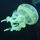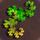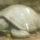## General Question# How do I do this math problem? (please see details)

Asked by NostalgicChills (2784) May 27th, 2012

My brother needs help with his homework and the material is stuff that I learned 4 years ago. That said, I don’t really remember it. I tried looking it up but I can’t seem to find what I need.

One of the questions has a picture of a graph with a line and two points. We figured out that the points are (1,4) and (2,6). The directions say to find the slope and y-intercept of the problem.
What now? Do we use the m=y2-y1/x2-x1 formula to find the slope? And what do we do to find the y-intercept?

Observing members: 0Composing members: 0You are correct in saying that the slope is given by the formula:
m = (y2-y1)/(x2-x1)
Depending on the situation, you might find this (only slightly different) formula easier:
m = (y1-y2)/(x1-x2)

For your problem, the slope would be m=(6–4)/(2–1)=2/1=2.

After you find the slope, finding the y-intercept is easy. Use the formula y=mx+b. This is called the slope-intercept form of a line, which gives the y-coordinate given the x-coordinate. The m is the slope, and the b is the y-intercept.

Substitute this formula with the y coordinate of either of the points and the x coordinate of the same point, along with the slope you found earlier.
4 = 2*1+b
b=2
This means that the y-intercept is 2.

You can verify this by graphing the line on graph paper.

PhiNotPi (12677)“Great Answer” (6) Flag as…@PhiNotPi Thank you so much!
I just have one more question: There are other multiple choice problems that give an equation and then gives you ordered pairs as choices for your answers. For example: 3x+4y=16
I know the answer is B.) (4,1) but how can he show the actual work for it?

NostalgicChills (2784)“Great Answer” (0) Flag as…Some other technical notes (that I don’t like) is that the y-intercept should always be written in the form of an ordered pair (0,x). In this case, you should only put (0,2). A math teacher is unlikely to accept anything else.

For your second question, the only way to solve it is to simply find out which point is on the line. It is impossible to solve (there are an infinite number of points on a line) given just the question. That is why the question limits it to four or five possibilities! The only way to solve this is to substitute the x- and y-coordinates of each answer into the equation and see if they work.

For your example of 3x+4y=16 and the answer choice of (4,1), you would substitute 3*4+4*1=16, which is a true statement, so you know that it is the correct answer choice.

PhiNotPi (12677)“Great Answer” (4) Flag as…@PhiNotPi Thank you, again!

NostalgicChills (2784)“Great Answer” (0) Flag as…I know this is an old question, but doing math like this via “which formula should I use” bothers me a bit. Not that its your fault… the way we teach math in schools is horrible.

sorry, pet peeve

roundsquare (5517)“Great Answer” (0) Flag as…@roundsquare I agree. Simply memorizing the formula does not mean that you understand the math. You have to learn why the formula works. If a person knows why the math works, then he is able to create new formulas and to better use them.

Here’s one example:
Area of regular polygon = ½ * apothem * perimeter
Regardless of number of sides.

That is one simple formula that is very useful in a geometry class, but I don’t know if anyone realizes how this equation works. It is derived by dissecting the regular polygon with N sides into N congruent triangles, each with its base as a side of the polygon. The formula for each triangle is (Apothem * Side Length / 2). The total area is then (N * (Apothem * Side Length / 2)). From this, the (N * Side Length) = Perimenter, so the formula then simplifies to the above.

Not understanding shortcuts is another reason why some people find math harder than it should be. I have witnessed people pull out a calculator to divide by 1000. If a person understood the trick (move the decimal point to the left three spaces, which is the number of zeros in the number), then they could do the problem without any real effort.

Very few people understand how the quadratic formula works. The fact that there is a quadratic formula “song” shows how we are concerned with memorization. In 8th grade, I managed to (re-)derive the quadratic formula from scratch.

@NostalgicChills Sorry for getting off topic.

PhiNotPi (12677)“Great Answer” (0) Flag as…@PhiNotPi I never saw that apothem formula before, but I love it. Very elegant.

The quadratic formula is probably the best example. I was annoyed that my sister’s teacher didn’t teach it conceptually at all so I retaught it to her as “move that annoying constant term to the other side and then figure out what you need in order to get something that squares on the left.”

Another example from chemistry is the formula PV = nRT. My teacher wanted us to remember it as “pee-vee equals nert.” I really hated that.

I’d be much happier if most kids only got through algebra 1 and/or geometry but really understood it. I had a math professor once who told us that in the 1700s (I think) college level math was adding fractions but they made sure the concept was understood.

roundsquare (5517)“Great Answer” (0) Flag as…or